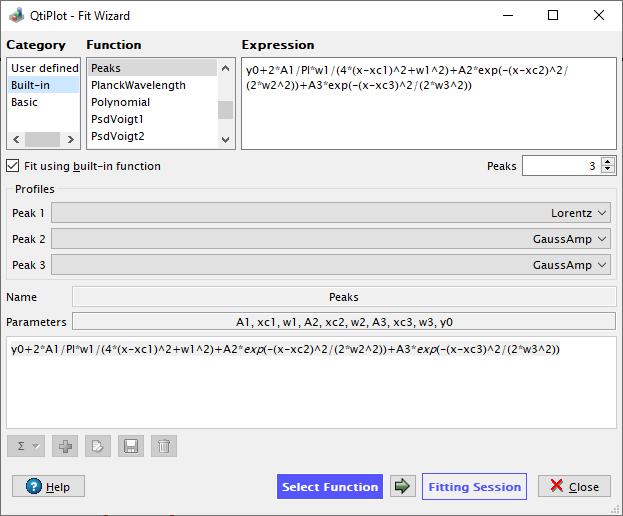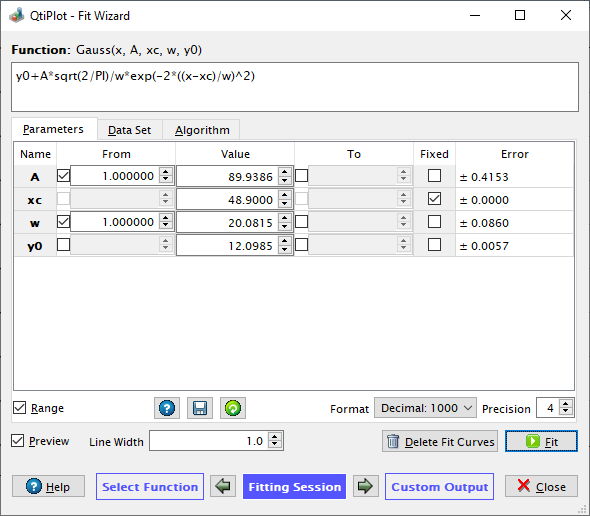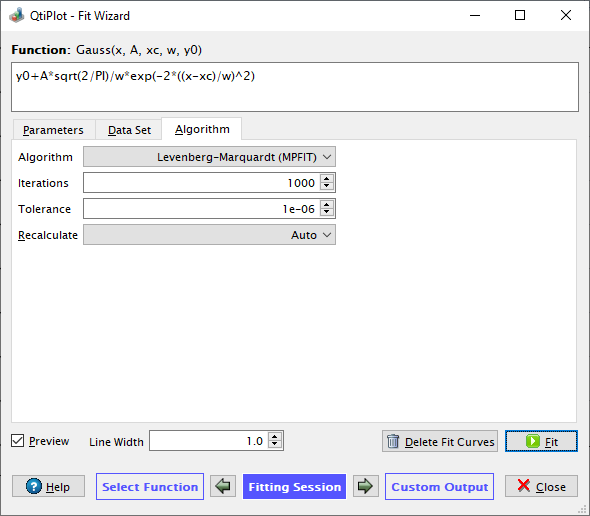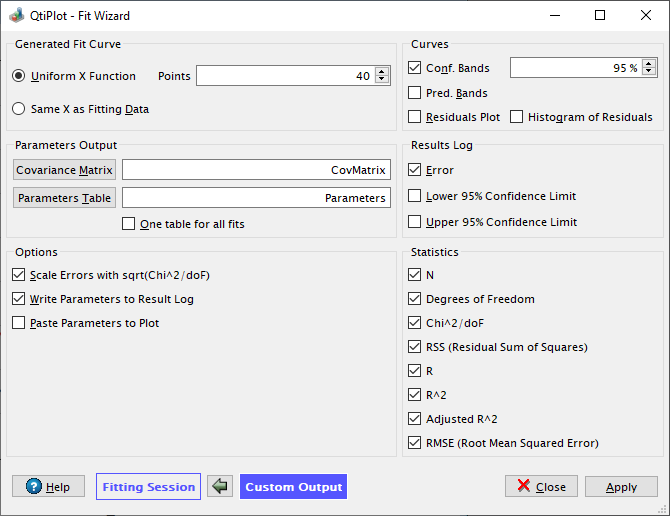# The Fit Wizard

This dialog is activated by selecting the command Fit Wizard... from the Fitting -> Analysis menu. This command is active if a plot or a table window is selected. In the latter case, this command first creates a new plot window using the list of selected columns in the table.

This dialog is used to fit discrete data points with a mathematical function. The fitting is done by minimizing the least square difference between the data points and the Y values of the function, see the Data Fitting section of the Analysis chapter for more details.

## Select Function Tab

The top of the dialog box is used to select one of the four Categories of functions:

User defined:

functions which have been previously edited and saved by the user.

Built-in:

the fit models provided by QtiPlot in the from the Fitting -> Analysis menu.

Basic:

simple elementary functions.

Plugins:

external functions provided via plugins.

To choose a function, first select a category and then the desired function from the displayed Function list.

If the selected function is from the Built-in category and you wish to perform the fit operation using the selected built-in model, you must check the option Fit using built-in function. This will clear the contents of the edit text pane (see image below) and copy the selected function into it.

If the selected built-in model is one of the following peak shape functions: Gauss, Lorentz, PsdVoigt1 or PsdVoigt2, the Peaks input box becomes available, allowing to choose the number of individual peaks for a multi-peak fit operation.

When the selected built-in model is the Polynomial function the Polynomial order input box becomes available, allowing to choose the order of the polynomial fit function.

If you wish to define your own fit model, the Fit using built-in function option should be unchecked. In this case you can also click the Add expression button to copy the selected function, but this will not clear any previous contents from the edit text pane. If you've selected one of the Basic functions, there are no checkboxes/options available and you need to use the Add expression button.

The bottom half of the dialog box allows you to define your own function. You can either write you own mathematical expression from scratch or add expressions from the function selector with the Add expression button. Once a custom expression is completed, clicking on the Save button will add the function to the list of user defined functions. The Name field will be used as the name of the function. A copy of the function is saved on disk with the extension ".fit". You can define the folder where ".fit" files are saved using either the Choose models folder... button (shown only when "User defined" is selected) or by selecting a new folder in the Save file dialog. Functions can be removed from the User Defined list by selecting them and clicking on the Remove button. You will be asked to confirm the deletion.

Figure 5-125. The first step of the Fit Wizard... dialog box.

This first step is used to define the function which will be used for fitting. When you are ready to perform the fit, click on thebutton to activate the Fitting Session.## Fitting Session Tab

In this second step the parameters of the fit must be defined. A starting point must be provided for the fitting parameters usig the Initial guesses tab.

Figure 5-126. The Fit Wizard... dialog box: providing initial guesses for the fit parameters.In the Data Set tab you can choose the input data set(s) and also choose a weighting method for your fit (the default is No weighting). The available weighting methods are:

1. No weight: all weighting coefficients are set to 1 (wi = 1).

2. Instrumental: the values of the associated error bars are used as weighting coefficients wi = 1/eri2, where eri are the error bar sizes stored in error bar columns. You must add error bars to the analyzed curve before performing the fit.

3. Statistical: the weighting coefficients are calculated as wi = 1/yi, where yi are the y values in the fitted data set.

4. Arbitrary Dataset: allows setting the weighting coefficients using an arbitrary data set wi = 1/ci2, where ci are the values in the arbitrary data set. The column used for the weighting must have a number of rows equal to the number of points in the fitted curve.

5. Direct Weighting: allows setting the weighting coefficients using an arbitrary data set wi = ci, where ci are the values in the arbitrary data set. The column used for the weighting must have a number of rows equal to the number of points in the fitted curve.

Figure 5-127. The Fit Wizard... dialog box: choosing the input data set(s).It is also possible to specify how the fit operation responds to modifications of the source data curve using the Recalculate list box. The available options are:

No

- No recalculation when data changes, the fit object is deleted from memory when the operation ends.

Auto

- A recalculation is automatically performed when input data changes.

Manual

- No recalculation is triggered when data changes, instead you can perform a recalculation at any time by pressing the Recalculate button from the Analysis tab of the plot details dialog.

The recalculation mode can be changed later on via the Analysis tab of the plot details dialog.

Figure 5-128. The Fit Wizard... dialog box: choosing the fit algorithm.## Custom Output Tab

Depending on the settings in the Custom Output tab, a function curve (option Uniform X Function) or a new table (if you choose the option Same X as Fitting Data) will be created for each fit. The new table includes all the X and Y values used to compute and to plot the fitted function and is hidden by default. It can be found in and viewed from the project explorer.

After the fit, if the Write Parameters to Result Log box from the Options group is checked, the log window is opened to show the results of the fitting process.

Figure 5-129. The third step of the Fit Wizard... dialog box.

This third step is used to customize the output of a fitting operation.The options in the Curves group box can be used to specify if some additional plot curves should be generated after each fit operation. If the Conf. Bands and Pred. Bands options are checked QtiPlot generates confidence bands and prediction bands for the current fit, based on the user defined value for the confidence level. If the Residuals Plot option is checked the curve of the fit residuals is also displayed. The residuals are calculated as the difference between the experimental Yi values and the response values predicted by the fit model.

The controls in the Parameters Output group box can be used in order to define the options for the display of the results from data fit operations. The Format list box allows to choose a default numerical format. The value of the Significant Digits option can be used to customize the precision of the output and has a different meaning depending on the numeric format. The following format and precision options are available:

Default

- Decimal or scientific e-notation, whichever is the most concise. The value of the Significant Digits control represents the maximum number of significant figures in the output (trailing zeroes are omitted).

Decimal: 1000

- Decimal notation. The value of the Significant Digits control represents the number of digits after the decimal point.

Scientific: 1E3

- Scientific e-notation where the letter `e` is used to represent "times ten raised to the power of" and is followed by the value of the exponent. The value of the Significant Digits control represents the number of digits after the decimal point.

### Reported errors

The errors on the fit parameters are displayed in the log window only if the Error box from the Results Log group is checked. The Scale Errors with sqrt(Chi^2/doF) option only affects the errors on the parameters reported after the fit operation. It does not affect the fitting process or the data in any way. If checked, the reported errors on the parameters, εj, are calculated as the square root of the diagonal elements of the covariance matrix Cov(j, j) multiplied with sqrt(Chi2/(n - p)), where n is the number of data points and p the number of fit parameters. Otherwise, the reported errors equal the square root of the diagonal elements of the covariance matrix Cov(j, j).

If the LCL and UCL options from the Results Log group box are checked QtiPlot also displays the confidence limits for each fit parameter based on the value of the confidence level specified in the Curves box.

### Goodness-of-Fit Statistics

After the fit, a series of statistics are displayed in the log window allowing evaluation of the goodness of fit. The controls in the Statistics group box can be used to specify which of these fit statistics should be displayed by default. For more details about these values please visit the Data fiting section from the chapter dedicated to the Analysis of data and curves.